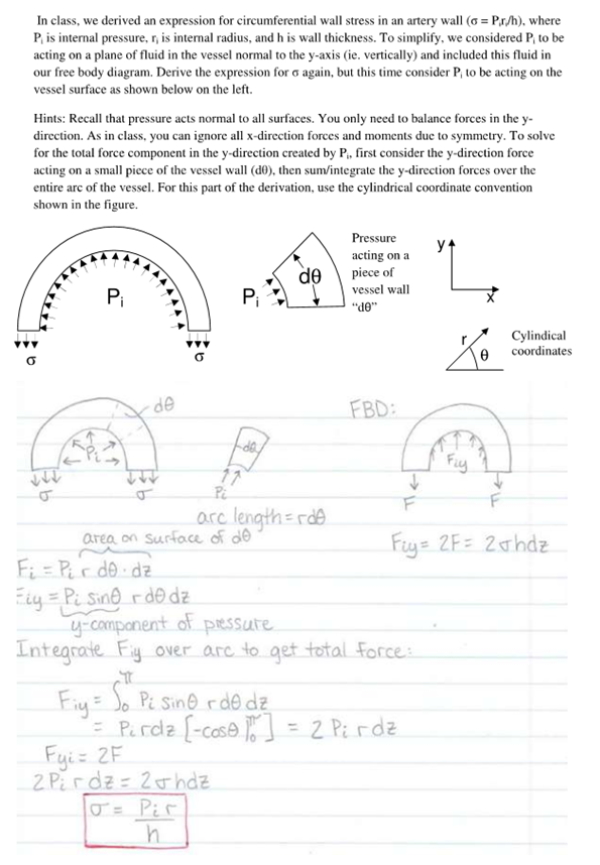In class, we derived an expression for circumferential wall stress in an artery wall, sigma=Pr/h, where Pi is internal pressure, r, is internal radius, and h is wall thickness. To simplify, we considered Pi to be acting on a plane of luid in the vessel normal to the y axis and included this fluid in our free body diagram. Derive the expression for sigma again, but this time consider Pi to be acting on the vessel surface as shown below on the left.In class, we derived an expression for circumferential wall stress in an artery wall, sigma=Pr/h, where Pi is internal pressure, r, is internal radius, and h is wall thickness. To simplify, we considered Pi to be acting on a plane of luid in the vessel normal to the y axis and included this fluid in our free body diagram. Derive the expression for sigma again, but this time consider Pi to be acting on the vessel surface as shown below on the left.

biomechanics page 1 biomechanics biomechanics biomechanics biomechanics biomechanics biomechanics biomechanics biomechanics page 2